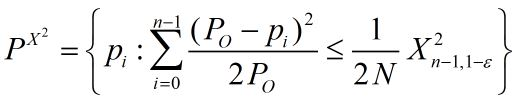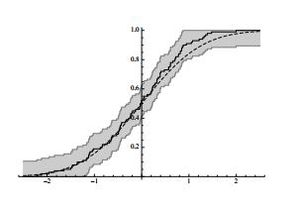# Data driven robust optimization

Author: Watson Fu (ChE 345 Spring 2015)

Steward: Dajun Yue, Fengqi You

# Background

Robust optimization is a distinct approach to optimizations problems that allows for the incorporation of uncertainty. The usefulness of robust optimization lies in the ability to solve for every realization of the uncertain parameters. As a result, the problem can be solved for the worst-case scenarios of the entire set of uncertainty.1 The most vital aspect of robust optimization is the determination of the uncertainty set. As an uncertainty set grows, it will be able to undertake more realizations. The drawback from assuming a large uncertainty set is the concern of the overall optimization problem becoming computationally intractable. At the same time, a small uncertainty set will yield an answer that is conservative and ignorant of aspects of the uncertainty.2 The general concepts and usage of robust optimization are now being shifted because of the availability and shear volume of data for every aspect of life.3

From complex supply chains to internet user preferences, these data is forcing change in how robust optimization problems are being approached. The existence of these data can eliminate the need for unproven assumptions and reasoning, which were previously needed in many robust optimization problems in order to make the problems tractable.2

# Description

Data-driven optimization has already been studied and implemented in various systems, but many of these studies are divergent. The basic principle that ties together varying applications of data-driven optimization is the concept of defining uncertainty through data analysis. For example, take a particular parameter or set, $\mathbf {u}$with uncertainty. This set, $\mathbf {u}$will be defined as a random variable with probability distribution $P$. Data-driven optimization is centered around using pertinent data and analysis of data to help define the probability distribution $P$.3 If the probability distribution of a certain random variable can be realized, it can lead to a more complex analysis of a given problem.

# Methodology and Formulation

The crux of data-driven robust optimization is the incorporation of hypothesis testing and statistical methods to help design uncertainty sets. 4. The basic definition of the problem can be seen in this linear constraint:

$\mathbf {u} ^{T}x\leq b\quad \forall \mathbf {u} \in U$The constraint $\mathbf {u}$is a robust constraint because it is uncertain. As mentioned before, the probability distribution of $\mathbf {u}$will be called $P$and it is unknown and the focus of any data-driven robust optimization problem. It is also important to define a probability, $\delta$, for a particular set $U$:

$P(\mathbf {u} ^{T}x\leq b\quad \forall \mathbf {u} )\geq 1-\delta$This statement defines the probability $\delta$as the probabilistic guarantee with respect to the given probability distribution $P$with level $\delta$.

These definitions are part of every instance of data-driven robust optimization, but the design of the uncertainty set and the probability distribution is different. These nuanced differences in the specific design of the sets can be changed depending on the data available and the characteristics of the optimization problem.

## Different Constructions of Uncertainty Sets

The choice of construction of the uncertainty set is dependent on data available and properties of the probability distribution. Each construction involves some hypothesis testing on the probability distribution $P$. It is useful to have a basic understanding of statistical hypothesis testing.

### Uncertainty Sets from Discrete Distributions

In this specific definition of the uncertainty set, the main assumption is that the probability distribution $P$has a finite support that is known. For example, a Bernoulli distribution or a binomial distribution are common probability distributions with finite supports. This means that the set of probability is not zero-valued and can be discretized.

As a result, it is possible to use the Pearson's $X^{2}$test to aid in the construction of a viable probability distribution. In the Pearson's $X^{2}$test, a null hypothesis of $P=P_{O}$is defined.

Next, define a empirical probability distribution over the sample $p_{i}$, and a confidence level $\epsilon$at which the null hypothesis will be rejected.

It follows that the confidence region, by this definition, will be.

Consequently, the original set of uncertain parameters, $U$can be redefined as only containing the constraints whose probability distributions fall in the acceptable range $\epsilon$. This will redefine the uncertainty set and give a more relaxed feasible region, but maximizing the confidence in any optimal solution.

### Uncertainty Sets from Independent Marginal Distributions

This construction of the uncertainty set is used for a probability distribution that has a continuous support. The marginal distribution $P^{*}$of any probability distribution $P_{O}$is the a certain subset that holds a specific probability that does not reference the other values of the set not accounted for in the particular subset. The reason why it is beneficial to consider independent marginal distributions when analyzing a probability distribution with a continuous support is because multivariate fit testing has not reached the capacity to holistically analyze the problem.5 Another common way to approach this problem is to discretize the continuous function so that the support now becomes a discrete function.

#### Utilization of Kolmogorov-Smirnov TestDevelopment of a confidence region from an arbitrary function based on a Kolmogorov-Smirnov Test4

The Kolmogorov-Smirnov test is a generic fit test that will test two continuous samples for equality. This test will be applied in the same fashion as the Pearson's $X^{2}$test was utilized for the discrete variable case. It is important to note that there are some limitations to this model can be seen in its formulation because it is mostly utilized for univariate cases.4 This construction requires finding a confidence region for a certain marginal distribution $P^{*}$within the probability distribution $P$. There also needs to be a defined $\epsilon$at which the null hypothesis that $P^{*}$will be rejected. The use of the Kolmogorov-Smirnov test can grant a defined uncertainty set $U$, but it has the drawback of becoming ineffective at solving multivariate problems. As a result, sometimes data-driven robust optimization problems where the probability distribution of the uncertainty set has a continuous support are solved by converting it to a discrete support and solving by the aforementioned techniques for discrete distributions.5

# Applications

## Applications in Chemical Engineering

Data-driven robust optimization is useful in chemical engineering because of the complexity of design problems. As a result, it can utilize characteristic properties of systematically collected data to optimize certain features of any given system. For example, data-driven robust optimization can be used in complex supply chain optimization problems. The benefit of data-driven robust optimization for supply chain problems is that behavior of suppliers and consumers can be modeled through historical demand data, and the data associated with past decisions.6

In chemical engineering, various operations and reactor systems can also be optimized through data-driven robust optimization. For these problems, data-driven robust optimization can aid in the isolation of complex relationships between given parameters. More importantly, these concepts can be used in a dynamic fashion in combination with various design of experiment techniques.7

### Example: Reactor Design Optimization

Because of the nature of data-driven robust optimization, it requires large amounts of data, even for simple problems. Therefore, a case study for the robust optimization of a simple reversible reaction in batch reactor is presented.7 This is a given reaction for reactant A to product B. The goal of the case study is to maximize the production of B by modeling the problem and applying data-driven robust optimization techniques based on experimental results to try to reach a maximum.

The problem is modeled with certain set set of differential equations, and is constrained by physical requirements. The most notable aspect of this case study is how they implement data-driven optimization. The define an additional constraint of error based on defined factor to temperture. These factors effect is refined through ANOVA (Analysis of variance) and the error is made into a response surface model, and this is reevaluated in iterations of optimization.

## Applications in Finance

This form of robust optimization is also useful in portfolio management. While it is an inherent feature in data-driven robust optimization, variance analysis is also the focal point of portfolio management. The idea for any portfolio management approach is centered around maximizing returns while minimizing a risk function for that target return. Data-driven optimization allows for the combination of this generic approach with relationships between stocks and presents a far more robust solution. As an extension, variance and covariance matrices can also be analyzed through data-driven robust optimization.8

# Conclusion

An important distinction between data-driven robust optimization and many other optimization problem is that it makes a probabilistic guarantee as opposed to making a claim about a deterministic optimal solution. This property of data-driven optimization yields applications in various fields.

Optimization is frequently shaped by a priori assumptions and reasonable estimates about certain features to simplify a problem.2 With data-driven optimization, these assumptions can be limited by data and statistical analysis that will give a more insightful solution. As more data becomes available, data-driven robust optimization will yield more precise and more illuminating solutions.